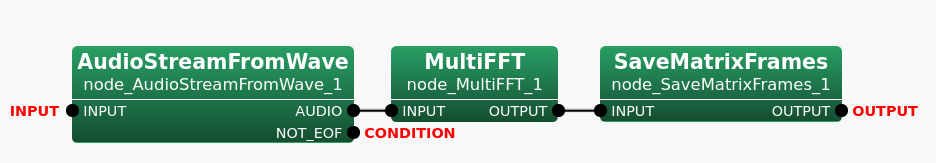## 6.7.27 SaveMatrixFrames

### 6.7.27.1 Outline of the node

Saves data of frames in the Matrix<ObjectRef>  type in a file. Note that the supported ObjectRef are the float type and the complex<float> type only.

### 6.7.27.2 Necessary file

No files are required.

### 6.7.27.3 Usage

When to use

This node is used to save data stored in the Matrix<ObjectRef>  type for multiple frames in one file.

Typical connection

Figure 6.134 below shows an example network using SaveMatrixFrames where MultiFFT outputs data of frames in the Matrix<complex<float> >  type.Figure 6.134: Connection example of SaveMatrixFrames

### 6.7.27.4 Input-output and property of node

Input

INPUT

: Data of frames in the Matrix<ObjectRef> type. The supported types are the Matrix<float>  type and the Matrix<complex<float> >  type.

Output

OUTPUT

: A file in which data in the Matrix<ObjectRef>  type for multiple frames were saved.

Parameter

Table 6.128: Parameter list of SaveMatrixFrames
 Parameter name Type Default value Unit Description FILENAME The file name of the output file. OUTPUTTYPE TEXT The file format options for the output file. Select TEXT for a text file or RAW for a binary file. TEXTFORMAT FIXED The file format options when the OUTPUTTYPE is set to TEXT. Select FIXED or SCIENTIFIC.
FILENAME

: string  type. The file name for the output file.

OUTPUTTYPE

: string  type. The file format options for the output file. Select TEXT for text files or RAW for binary files. The default value is TEXT.

TEXTFORMAT

: string  type. The file format options when the OUTPUTTYPE is set to TEXT. Select FIXED or SCIENTIFIC.

### 6.7.27.5 Details of the node

SaveMatrixFrames takes data in either the Matrix<float> type or the Matrix<complex<float> > type for multiple frames as the input and then saves all data in one file. The file format is to be specified in the OUTPUTTYPE parameter. When the OUTPUTTYPE parameter is set to RAW, data of frames will be written in IEEE 754 32-bit single-precision floating-point number format by little- endian order. The default value of this parameter, TEXT, will output human-readable data unlike RAW.

Table 6.129 shows the details of how data will be formatted in the file when TEXT is selected in the OUTPUTTYPE parameter. Each data is separated by space and the data of frames are separated per frame in the size of the Matrix by a line feed.

Table 6.129: File Format for TEXT OUTPUTTYPE
 Datatype File Format Float data data data data ... data[m][n] data data data data ... data[m][n] Complex data.real() data.imag() data.real() data.imag() ... data[m][n].real() data[m][n].imag() float

Where n and m are the column size and the row size of the Matrix for the input in which data of frames were stored.

SaveMatrixFrames checks the size of rows and columns of the Matrix when it receives the data for each frame. In the sample network above (Figure 6.134), MultiFFT outputs Matrix<complex<float> > . When the input matrix size is M x L, the output matrix size is M x L/2+1 for MultiFFT where M stands for the number of channels and L stands for the sample number of waveforms. Window Length in MultiFFT is 512 by default. Applying the formula for L will give 257 for the column size. When the number of channels is 8, the row size will be 8. For more details on MultiFFT output, refer to MultiFFT Node Reference.

Suppose the input data were stored in the Matrix<float> type where the column size is 512 and row size is 8, and there are 6397 frames in total, the contents of the output file will be like one shown in Figure 6.135. It is assumed that the data were saved in the TEXT file format described in the Table 6.129.Figure 6.135: Sample contents of the output data file in the TEXT file format where the data are in the float type

Note that the Frame numbers written on the left side of Figure 6.135 and Figure 6.136 are not written in the files.

Figure 6.136 gives sample contents of the output file when the input data were in the Matrix<complex<float> > type like ones in the sample network given by Figure 6.134. The row size and the column size are 8 and 257, respectively. There are 6397 frames in total. It is also assumed that the data were saved in the TEXT file format described in the Table 6.129.Figure 6.136: Sample SaveMatrixFrames Output File Content for TEXT Complex Float Type

Filename:

An option to use a special pattern, {tag:format}, with the data properties as known as a format string is available when specifying the value in the FILENAME parameter. The format string allows the users to set a filename which contains the information of the input data and so convenient for the later use. The available tags are listed in the Table 6.130.

Table 6.130: Tag list of SaveMatrixFrames
 Tag Description Unit datatype Data Type (float or complex float) String rowsize Row size of Matrix Integer colsize Column size of Matrix Integer dim Data Dimension (Fixed value. 2 for Matrix) Integer

The examples of the format string and the output are given in the Table 6.131.

Table 6.131: Examples of the format string and the output for Matrix<complex<float> > where the row size is 8 and the column size is 512
 Format String (FILENAME Parameter value) Output (Formatted Filename) samplefile.dat samplefile.dat samplefile_{datatype}.txt samplefile_complex_float.txt samplefile_row{rowsize}.raw samplefile_row8.raw samplefile_col{colsize}.dat samplefile_col512.dat samplefile_dim{dim}.dat samplefile_dim2.dat samplefile_{datatype}_row{rowsize}_col{colsize}_dim{dim}.dat samplefile_complex_float_row8_col512_dim2.dat

It is required to specify the column size, the row size, and the data type in parameters when using LoadMatrixFrames to load a file outputted by SaveMatrixFrames into the Matrix<ObjectRef> type. Hence, having data information in the filename will be useful for the later use.

As indicated in the Table 6.131, there is no rules on the file name extension. It can be any as user specify. It allows to have no file name extensions as well.

The value for "format" in {tag:format} is optional. The "format" can be used to specify the number of digits, for instance, 03d where the format specifiers are the same as the ones of printf in C.